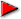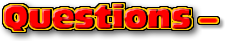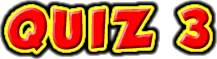ChaptersPage 3This text is meant to accompany class discussions. It is not everything there is to know about uniform circular motion. It is meant as a  prep for class. More detailed notes and examples are given in the class notes, presentations, and demonstrations (click here.)Click for the questions that go with this reading
Solving Word Problems in Kinematics

A big part of any physics class is spent solving word problems. This section will show you how to approach these problems.

Know your concepts. Later this will help you to identify what concepts are applicable. These concepts are often presented as variables in math models. You need to be able to identify what you are given in word problem. The first clue can be found in the units.

Physics has a set of units called fundamental units. These are units that cannot be described using other units. In kinematics these units are time and distance. Some variables in kinematics can be identified by the fundamental units while others are identified by the combination of the units. Below is a list of variables and their corresponding units.If you see a number and unit combination like 4 m/s2 in a word problem you can recognize the 4 as an acceleration. This is because the units are a distance divided by two time units. If you see a unit like 6 m/s in a word problem, then you would recognize the number 6 a velocity because the units are a distance unit divided by a single time unit. But you do not know if this is an initial, final, or an average velocity. This piece of information is fond by reading the word problem as if it were a story. In the story does the 6 m/s occur at the beginning or the end. If you are lucky the word problem tells you which velocity by saying something like, "...the average velocity is 6 m/s."There are some variable that are implied either by a word choice or by the situation. The words "stop" and "rest" mean that the velocity of an object is zero. For example, "A car rolls to a stop..." means that the final velocity is zero. "A runner start from rest before ..." This implies that the initial velocity is equal to zero. Another implied given comes from the word "drop." This implies two givens; the initial velocity is zero and the object will accelerate downwards at 9.80 m/s2 near the Earth's surface. "Iron man stood on top of a tall building and dropped a car to the ground below." This implies that vo = 0 and a = 9.80m/s2 downwards

 word or phrase implied given stop a velocity is zero rest a velocity is zero drop the initial velocity is zero AND the object will accelerate downwards at 9.80 m/s2. constant velocity the acceleration is zero

 Example #1 (Listing the givens) List the givens for this word problem, "While at a baseball game, a boy dropped form the upper deck to the deck 8.00 m below. What velocity did the boy hit the deck with?" List the givens for this problem. (Do not solve.) A single "given" must have at least has three parts: variable, number with a sign, and units. Listing the "givens" is the first step in solving a problem. It allows you to see what you have and what you need. Never skip this step. Solution:This is what you paper looks like:Example #2 (Listing the givens) A car starts from rest and accelerates to 22 m/s in 13m. What was the car's acceleration? List the givens for this problem. (Do not solve.) Solution:This is what you paper looks like:Example #3 (Listing the givens) A skateboarder is moving at 4.50 m/s when he begins to slow down by dragging his feet. He reaches a speed of 1.00 m/s in 5 seconds. How much distance did this journey take? List the givens for this problem. (Do not solve.) Solution:This is what you paper looks like:Hint: Every acceleration will get its own list of givens. The beginners problems will only have one acceleration. Later the problems will change and have segments with different constant accelerations. This will require several lists of givens.

 When are Numbers Negative in the Equations? In kinematics and dynamics, the negative sign means the opposite direction of what you, the reader assume to be positive. To make it easier for us to compare solutions we will assume measurements pointing "down" or "downwards" are negative in our formulas. The acceleration due to gravity is always negative. When a problem describes the motion of an object as slowing down or describes it initial velocity as being larger then it final velocity, the the acceleration is negative. When the velocity points downwards it is negative. Either the word problem will describe this by using the words, "down," or, "downwards," or from the description you can determine that the object is moving downwards. The displacement, "x," is negative when a object lands lower than it is started from.
Example #4 (Choosing the Signs)
A ball is tossed upwards from the roof of a tall building. On the way down the ball misses the edge of the building and continues downwards until it hits the ground. What are the signs of the variables?
Solution
 xO 0 ...Nearly always for us x - The displacement between where the motion started and stopped is from the roof to the ground. This is downwards. vO + The object is tossed up initially. v - When the object lands it is traveling down, into the ground. t + ...Always a - It's velocity changes due to gravity. Therefore its acceleration is negative, downwards.Example #5 (Choosing the Signs)
A ball is thrown down with a velocity of 20 m/s from the roof of a tall building. The building is 37 m tall. Eventually the ball will hit the ground. What are the signs of the variables?
Solution
 xO 0 ...Nearly always for us x - The displacement between where the motion started and stopped is from the roof to the ground. This is downwards. Numerically it is, "-37m." vO - The problem states that the ball is thrown "down" with a velocity of 20 m/s. The unit clue tells me this is a velocity. This velocity happens at the beginning of the motion -that makes it vO. The word "Down" means that this velocity is negative. v - When the object lands it is traveling down, into the ground. (It is not zero until AFTER it hits the ground. We want to know about "when" it hits the ground. t + ...Always a - It's velocity changes due to gravity. Therefore its acceleration is negative, downwards. In fact it is -9.80 m/s2. For us, the value of gravity will nearly always be zero. There are exceptions to this rule, but this will be covered later.Reality Check. The fact that all the signs on the variables above are the same, really means that the signs on all of these numbers in the equations are THE SAME.

Example #6 (Choosing the Signs)
A car is traveling along a road at 15 m/s. It then slows down at a rate of 2.5 m/s2 to 5 m/s...
Solution
 xO 0 ...Nearly always for us x + The displacement, "x," is not described with any direction -make it positive. vO + The INITIAL velocity does not give any indication of direction -make it positive. Its value is 15 m/s. v + The FINAL velocity does not give any indication of direction -make it positive. Its value is 5 m/s. t + ...Always but it not needed or listed in this description. a - The car's motion starts at 15 m/s and then slows to 5 m/s. This means that is is slowing DOWN. When the word, "down," is used to describe the acceleration, it is negative. the value is -2.5 m/s2. (Notice that this value is not 9.80 m/s2. That is because gravity is not moving the car.)Putting it all together

At this point you should be able to list the givens in a simple kinematics problem. The next step is to use these given to solve. There are two ways to solve a word problem. the first method is to use concepts and logic. The second is to use the physics equations. The concept method will be focused on in class. Here is the equation method.

For kinematics, you should have memorized these four equations.This example video will show the steps for solving a basic word problem in kinematics as well as what I'm looking for for complete credit. When looking at the two example videos below, pay attention to how the correct math equation is selected. (This has not been described above.)

Example #7
• Question
• Video Solution
• Your paper
A meteor falls from the sky to the Earth. The meteor already had an initial velocity downward when it was spotted. If it hit the Earth at 335 m/s after being seen for 30 seconds, then what was the initial velocity of the meteor?
 THis video has a slight mistake. The final velocity is downward and should be negative. The video does not do this until the final math steps. This video can be found on YouTube at http://youtu.be/jihl63Apnfw

This is what you paper should look like. Notice that it includes a diagram illustrating the text, a list of givens showing numbers and units, a line of text describing what the formula is purporting to do, the equation with only variables, the equation with numbers and units, and the solution with units in a box so it can easily be seen.Example #8
• Question
• Hint
• Solution
• Your Paper
A car is slowing down from 22 m/s to a stop in 15 m. What is the car's acceleration?

A car is slowing down from 22 m/s to a stop in 15 m. What is the car's acceleration?This video can be found on YouTube at http://www.youtube.com/watch?v=qQ4zo4dFOaA

This is what your paper should look like when doing the work. This format will help you to clearly communicate your ideas and processes. Notice...

• A crude drawing with all the givens on it;
• the givens are neatly listed first;
• the givens include the variable, number, and units;
• the equations is next -it only contains the variables;
• the equation with numbers and units follows, and
• finally the answer with the units has a box around it to draw attention to it.Communication

Every scientist needs to be able to CLEARLY communicate to others the solutions to the problems he or she solves. There some parts to the solution that I'm always looking for. These parts will help you to organize your thoughts and to clearly communicate to others what you are doing.

In this image I annotated what you need to include to get complete credit for a solution.

 Part 1 A drawing with the variables you know and need to calculate are to be labeled on the diagram. Draw BIG. You do not need to be artistic. Below are four examples with various acceptable artistic skills.Part 2 A list of givens where each variable has a number AND unit. Be sure to include what variable you are looking for in the givens. Part 3 Equation used with only variables. Part 4 Rearrange the variable equation to isolate the unknown variable. (You can see how this is done in examples 7 & 8 above.) Part 5 Insert numbers WITH units in the rearranged equation. Part 6 Solution WITH correct unitsby Tony Wayne ...(If you are a teacher, please feel free to use these resources in your teaching.)